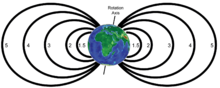# Dipole model of the Earth's magnetic field

﻿
Dipole model of the Earth's magnetic fieldPlot showing field lines (which, in three dimensions would describe "shells") for L-values 1.5, 2, 3, 4 and 5 using a dipole model of the Earth's magnetic field

The dipole model of the Earth's magnetic field is a first order approximation of the rather complex true Earth's magnetic field. Due to effects of the interplanetary magnetic field, and the solar wind, the dipole model is particularly inaccurate at high L-shells (e.g., above L=3), but may be a good approximation for lower L-shells. For more precise work, or for any work at higher L-shells, a more accurate model that incorporates solar effects, such as the Tsyganenko magnetic field model, is recommended.

## Equations

The following equations describe the dipole magnetic field.

First, define B0 as the mean value of the magnetic field at the magnetic equator on the Earth's surface. Typically$B_0=3.12\times10^{-5}\ \textrm{T}$.

Then, the radial and azimuthal fields can be described as$B_r = -2B_0\left(\frac{R_E}{r}\right)^3\cos\theta$$B_\theta = -B_0\left(\frac{R_E}{r}\right)^3\sin\theta$$|B| = B_0\left(\frac{R_E}{r}\right)^3 \sqrt{1 + 3\cos^2\theta}$

where RE is the mean radius of the Earth (approximately 6370 km), r is the radial distance from the center of the Earth (using the same units as used for RE), and θ is the azimuth measured from the north magnetic pole.

It is sometimes more convenient to express the magnetic field in terms of magnetic latitude and distance in earth radii. The magnetic latitude λ is measured northwards from the equator (analogous to geographic latitude) and is related to θ by λ = π / 2 − θ. In this case, the radial and azimuthal components of the magnetic field (the latter still in the θ direction, measured from the axis of the north pole) are given by$B_r = -\frac{2B_0}{R^3}\sin\lambda$$B_\theta = \frac{B_0}{R^3}\cos\lambda$$|B| = \frac{B_0}{R^3} \sqrt{1 + 3\sin^2\lambda}$

where R in this case has units of Earth radii (R = r / RE).

## Invariant latitude

Invariant latitude is a parameter that describes where a particular magnetic field line touches the surface of the Earth. It is given by$\Lambda = \arccos\left(\sqrt{1/L}\right)$

or$L = 1/\cos^2\left(\Lambda\right)$

where Λ is the invariant latitude and L is the L-shell describing the magnetic field line in question.

On the surface of the earth, the invariant latitude (Λ) is equal to the magnetic latitude (λ).

Wikimedia Foundation. 2010.

### Look at other dictionaries:

• Earth's magnetic field — Computer simulation of the Earth s field in a normal period between reversals. The tubes represent magnetic field lines, blue when the field points towards the center and yellow when away. The rotation axis of the Earth is centered and… …   Wikipedia

• Magnetic field — This article is about a scientific description of the magnetic influence of an electric current or magnetic material. For the physics of magnetic materials, see magnetism. For information about objects that create magnetic fields, see magnet. For …   Wikipedia

• Magnetic field of celestial bodies — See also Earth s magnetic field The magnetic field of a rotating body of conductive gas or liquid develops self amplifying electric currents, and thus a self generated magnetic field, due to a combination of differential rotation (different… …   Wikipedia

• Dipole — This article is about the electromagnetic phenomenon. For other uses, see dipole (disambiguation). The Earth s magnetic field, approximated as a magnetic dipole. However, the N and S (north and south) poles are labeled here geographically, which… …   Wikipedia

• Dipole (disambiguation) — In physics, a dipole is a quantity involved some form of polarity Electric dipole Magnetic dipole Dipole moment (disambiguation) In meteorology: Arctic dipole anomaly Indian Ocean Dipole It may also refer to: Dipole antenna, a type of radio… …   Wikipedia

• Earth Sciences — ▪ 2009 Introduction Geology and Geochemistry       The theme of the 33rd International Geological Congress, which was held in Norway in August 2008, was “Earth System Science: Foundation for Sustainable Development.” It was attended by nearly… …   Universalium

• Earth exploration — Introduction   the investigation of the surface of the Earth and of its interior.  By the beginning of the 20th century most of the Earth s surface had been explored, at least superficially, except for the Arctic and Antarctic regions. Today the… …   Universalium

• earth — /errth/, n. 1. (often cap.) the planet third in order from the sun, having an equatorial diameter of 7926 mi. (12,755 km) and a polar diameter of 7900 mi. (12,714 km), a mean distance from the sun of 92.9 million mi. (149.6 million km), and a… …   Universalium

• Magnetic resonance imaging — MRI redirects here. For other meanings of MRI or Mri, see MRI (disambiguation). Magnetic resonance imaging Intervention Sagittal MR image of the knee ICD 10 PCS B?3?ZZZ …   Wikipedia